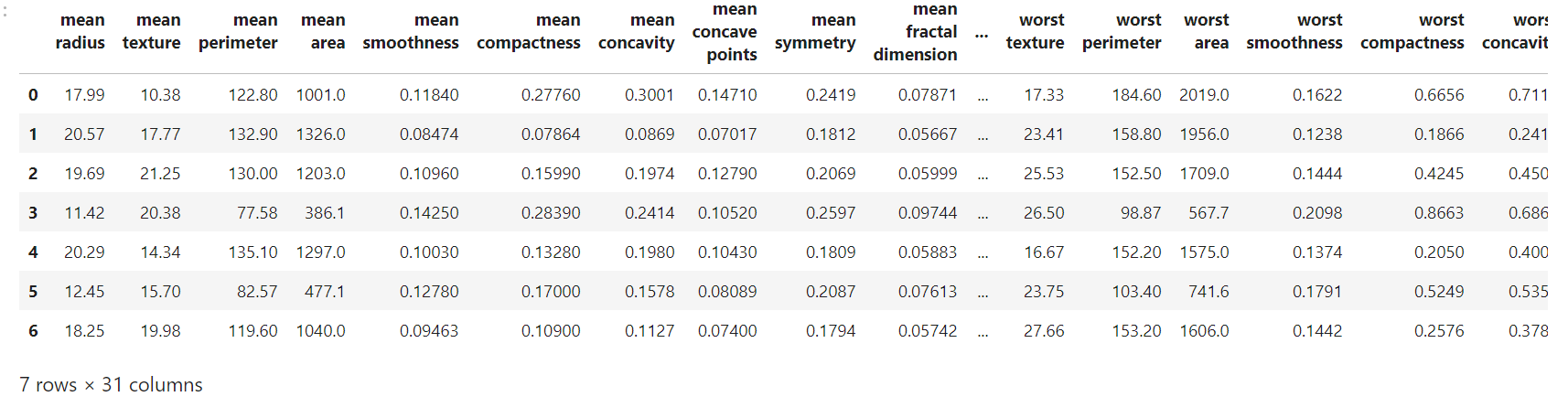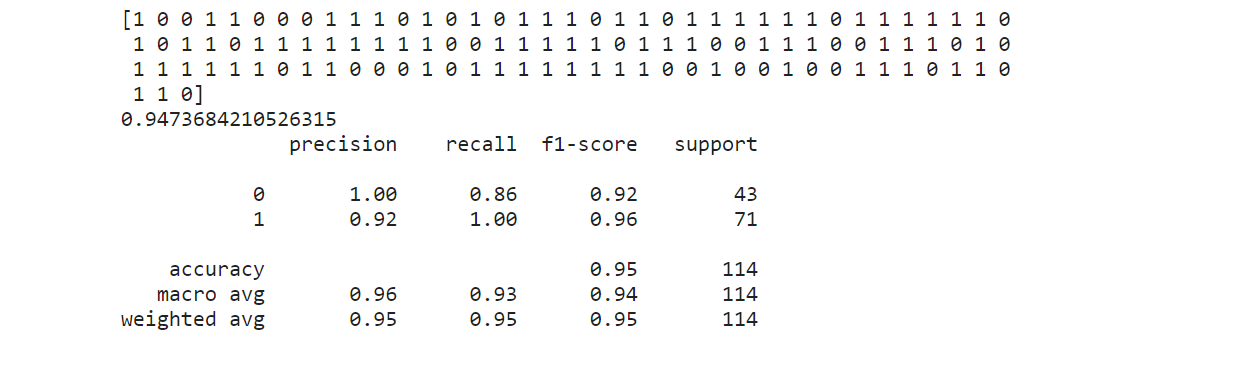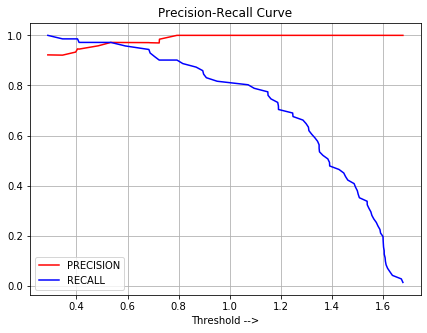# Decision Threshold In Machine Learning

What is Decision Threshold ?
sklearn does not let us set the decision threshold directly, but it gives us the access to decision scores ( Decision function o/p ) that is used to make the prediction. We can select the best score from decision function output and set it as Decision Threshold value and consider all those Decision score values which are less than this Decision Threshold as a negative class ( 0 ) and all those decision score values that are greater than this Decision Threshold value as a positive class ( 1 ).

Using Precision-Recall curve for various Decision Threshold values, we can select the best value for Decision Threshold such that it gives High Precision ( Without affection Recall much ) or High Recall ( Without affecting Precision much ) based on whether our project is precision-oriented or recall-oriented respectively.

The main purpose of doing this is to get a high precision ML model, or high recall ML model, based on whether our ML project is precision-oriented or recall-oriented respectively.

Code: Python code to build a high Precision ML model

 `# Import required modules. ` `import` `pandas as pd ` `import` `matplotlib.pyplot as plt ` `from` `sklearn ``import` `datasets ` `from` `sklearn.model_selection ``import` `train_test_split ` `from` `sklearn.svm ``import` `SVC ` `from` `sklearn.metrics ``import` `classification_report, recall_score, precision_score, accuracy_score ` ` `  `# Get the data. ` `data_set ``=` `datasets.load_breast_cancer() ` ` `  `# Get the data into an array form. ` `x ``=` `data_set.data        ``# Input feature x. ` `y ``=` `data_set.target      ``# Input target variable y. ` ` `  `# Get the names of the features. ` `feature_list ``=` `data_set.feature_names ` ` `  `# Convert the data into pandas data frame. ` `data_frame ``=` `pd.DataFrame(x, columns ``=` `feature_list) ` ` `  `# To insert an output column in data_frame. ` `data_frame.insert(``30``, ``'Outcome'``, y)       ``# Run this line only once for every new training. ` ` `  `# Data Frame. ` `data_frame.head(``7``) `

Output:Code: Train the model

 `# Train Test Split. ` `x_train, x_test, y_train, y_test ``=` `train_test_split(x, y, test_size ``=` `0.2``, random_state ``=` `42``) ` ` `  `# Create Classifier Object. ` `clf ``=` `SVC() ` `clf.fit(x_train, y_train) ` ` `  `# Use decision_function method. ` `decision_function ``=` `clf.decision_function(x_test) `

Actual Scores obtained:

 `# Actual obtained results without any manual setting of Decision Threshold. ` `predict_actual ``=` `clf.predict(x_test)       ``# Predict using classifier. ` `accuracy_actual ``=` `clf.score(x_test, y_test) ` `classification_report_actual ``=` `classification_report(y_test, predict_actual) ` `print``(predict_actual, accuracy_actual, classification_report_actual, sep ``=``'\n'``) `

Output:In the above classification report, we can see that our model precision value for (1) is 0.92 and recall value for (1) is 1.00. Since our goal in this article is to build a High-Precision ML model in predicting (1) without affecting Recall much, we need to manually select the best value of Decision Threshold value form the below Precision-Recall curve, so that we could increase the precision of this model.

Code:

 `# Plot Precision-Recall curve using sklearn. ` `from` `sklearn.metrics ``import` `precision_recall_curve ` `precision, recall, treshold ``=` `precision_recall_curve(y_test, decision_function) ` ` `  `# Plot the output. ` `plt.plot(treshold, precision[:``-``1``], c ``=``'r'``, label ``=``'PRECISION'``) ` `plt.plot(treshold, recall[:``-``1``], c ``=``'b'``, label ``=``'RECALL'``) ` `plt.grid() ` `plt.legend() ` `plt.title(``'Precision-Recall Curve'``)`

Output:Here in the above plot, we can see that if we want high precision value, then we need to increase the value of decision threshold     ( x-axis ), but which would decrease the value of recall ( which is not favourable). so we need to choose that value of Decision Threshold which would increase Precision but not much decrease in Recall. One such value form the above plot is around 0.6 Decision Threshold.

Code:

 `# Implementing main logic. ` ` `  `# Based on analysis of the Precision-Recall curve. ` `# Let Decision Threshold value be around 0.6... to get high Precision without affecting recall much. ` `# Desired results. ` ` `  `# Decision Function output for x_test. ` `df ``=` `clf.decision_function(x_test) ` ` `  `# Set the value of decision threshold. ` `decision_teshold ``=` `0.5914643767268305` ` `  `# Desired prediction to increase precision value. ` `desired_predict ``=``[] ` ` `  `# Iterate through each value of decision function output ` `# and if  decision score is > than Decision threshold then, ` `# append (1) to the empty list ( desired_prediction) else ` `# append (0). ` `for` `i ``in` `df: ` `    ``if` `i

Code: Comparison between old and new Precision Values.

 `# Comparison ` ` `  `# Old Precision Value ` `print``(``"old precision value:"``, precision_score(y_test, predict_actual)) ` `# New precision Value   ` `print``(``"new precision value:"``, precision_score(y_test, desired_predict)) `

Output:

```old precision value: 0.922077922077922
new precision value: 0.9714285714285714
```

OBSERVATIONS:

• The value of Precision has increased from 0.92 to 0.97.
• The Value of Recall has decreased due to Precision-Recall Trade off.

NOTE:
The above code is not data preprocessed( Data Cleaned or Feature Engineered ), which would makes this article prolonged. This is just an idea how to make use of Decision Threshold in practice.

My Personal Notes arrow_drop_upCheck out this Author's contributed articles.

If you like GeeksforGeeks and would like to contribute, you can also write an article using contribute.geeksforgeeks.org or mail your article to contribute@geeksforgeeks.org. See your article appearing on the GeeksforGeeks main page and help other Geeks.

Please Improve this article if you find anything incorrect by clicking on the "Improve Article" button below.

Article Tags :
Practice Tags :

2

Please write to us at contribute@geeksforgeeks.org to report any issue with the above content.